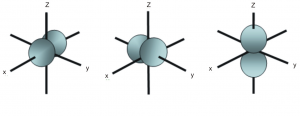Shapes of orbitals - Atomic orbitals

What are Atomic Orbitals?

Atomic orbitals are mathematical functions that describe the wave nature of electrons (or electron pairs) in an atom. They offer a way to calculate the probability of finding an electron in a specified region around the nucleus of the atom.

According to the quantum atomic model, an atom can have many possible numbers of orbitals. These orbitals can be categorized on the basis of their size, shape or orientation. A smaller sized orbital means there is a greater chance of getting an electron near the nucleus. The orbital wave function or ϕ is a mathematical function used for representing the coordinates of an electron. The square of the orbital wave function or represents the probability of finding an electron.

This wave function also helps us in drawing boundary surface diagrams. Boundary surface diagrams of the constant probability density for different orbitals help us understand the shape of orbitals. Let us represent the shapes of orbitals with the help of boundary surface diagrams:

The Shape of s Orbitals

The boundary surface diagram for the s orbital looks like a sphere having the nucleus as its centre which in two dimensions can be seen as a circle. Hence, we can say that s-orbitals are spherically symmetric having the probability of finding the electron at a given distance equal in all the directions. The size of the s orbital is also found to increase with the increase in the value of the principal quantum number (n), thus, 4s > 3s> 2s > 1s.The Shape of s Orbitals

The Shape of p Orbitals

Each p orbital consists of two sections better known as lobes which lie on either side of the plane passing through the nucleus. The three p orbitals differ in the way the lobes are oriented whereas they are identical in terms of size shape and energy. As the lobes lie along one of the x, y or z-axis, these three orbitals are given the designations 2px, 2py, and 2pz. Thus, we can say that there are three p orbitals whose axes are mutually perpendicular. Similar to s orbitals, size, and energy of p orbitals increase with an increase in the principal quantum number (4p > 3p > 2p).The Shape of p Orbitals

The Shape of d Orbitals

The magnetic orbital quantum number for d orbitals is given as (-2,-1,0, 1,2). Hence, we can say that there are five d-orbitals. These orbitals are designated as dxy, dyz, dxz, dx2–y 2 and dz2. Out of these five d orbitals, shapes of the first four d-orbitals are similar to each other, which is different from the dz2 orbital whereas the energy of all five d orbitals is the same.The Shape of d Orbitals